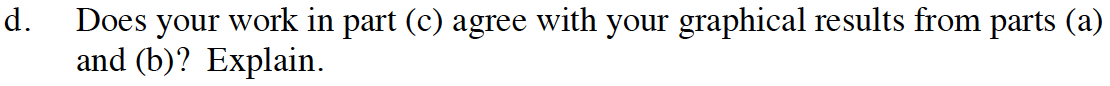Home > CCA2 > Chapter 12 > Lesson 12.2.3 > Problem12-133

12-133.
1. Consider the function f(x) = cos(x +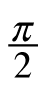). Homework Help ✎

1. Make a complete graph of f(x).

2. Write a simpler trig function that is represented by the same graph.

3. Now use the angle sum identity to expand and simplify cos(x +).

4. Does your work in part (c) agree with your graphical results from parts (a) and (b)? Explain.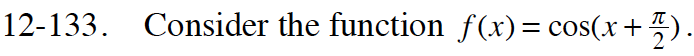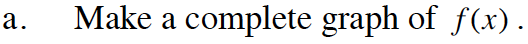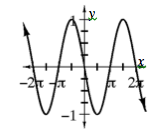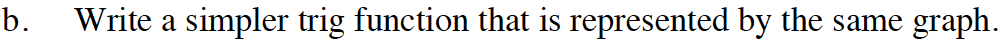Which trig function passes through the origin?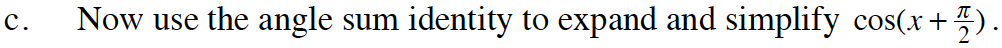Review the Math Notes box in this lesson and problem 12-130 for the Angle Sum Identities.